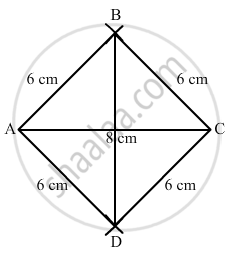Share

# Construct a rhombus with side 6 cm and one diagonal 8 cm. Measure the other diagonal. - Mathematics

Course
ConceptConstructing a Quadrilateral When Two Diagonals and Three Sides Are Given

#### Question

Construct a rhombus with side 6 cm and one diagonal 8 cm. Measure the other diagonal.

#### Solution$\text { Steps of construction: }$

$\text { Step 1: Draw AC = 8 cm } .$

$\text { Step 2: With A as the centre and radius = 6 cm, draw arcs on both sides } .$

$\text { Step 3: With C as the centre and radius = 6 cm, draw arcs on both sides, intersecting the previous arcs at points B and D }.$

$\text { Step 4: Join BD = 8 . 9cm }.$

$\text { Thus, ABCD is the required rhombus } .$

Is there an error in this question or solution?

#### APPEARS IN

RD Sharma Solution for Mathematics for Class 8 by R D Sharma (2019-2020 Session) (2017 to Current)
Chapter 18: Practical Geometry (Constructions)
Ex. 18.1 | Q: 5 | Page no. 4
Solution Construct a rhombus with side 6 cm and one diagonal 8 cm. Measure the other diagonal. Concept: Constructing a Quadrilateral - When Two Diagonals and Three Sides Are Given.
S